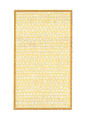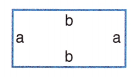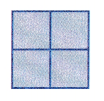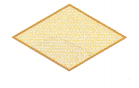Practice the questions of McGraw Hill Math Grade 8 Answer Key PDF Lesson 21.1 Quadrilaterals to secure good marks & knowledge in the exams.

Exercises

IDENTIFY

For each figure below, label as a square, rectangle, rhombus, trapezoid, or kite.

Question 1.Square,

Explanation:
We know if all the four sides are of the same length it is a square, as we have given shape have with all the four sides 4 in. so it is a square.

Question 2.Trapezoid,

Explanation:
As a trapezoid has two opposite sides that are parallel, but the sides are not the same
length and the other two sides of a trapezoid are not parallel, So given shape is trapezoid.

Question 3.Rectangle,

Explanation:
As the given shape has 2-dimensional figure with four right angles and the opposite sides are parallel and the same length so it is rectangle.

Question 4.Rectangle,

Explanation:
As the given shape has 2-dimensional figure with four right angles and the opposite sides are parallel with the same length a,a and b,bso it is rectangle.

Question 5.Trapezoid,

Explanation:
As a trapezoid has two opposite sides that are parallel, but the sides are not the same
length and the other two sides of a trapezoid are not parallel, So given shape is trapezoid.

Question 6.Square,

Explanation:
We know if all the four sides are of the same length it is a square, as we have given shape have with all the four sides equal so it is a square.

Question 7.Rhombus,

Explanation:
Given shape is rhombus with opposite sides are parallel and all the sides are the same length. Unlike a rectangle, a rhombus does not have four right angles.

Question 8.Kite,

Explanation:
Given shape is a kite as it is a quadrilateral which looks like a typical toy kite, so it is a kite, two of its angles are equal, Its longer two touching sides are equal in length and so
are shorter two touching sides.

Question 9.